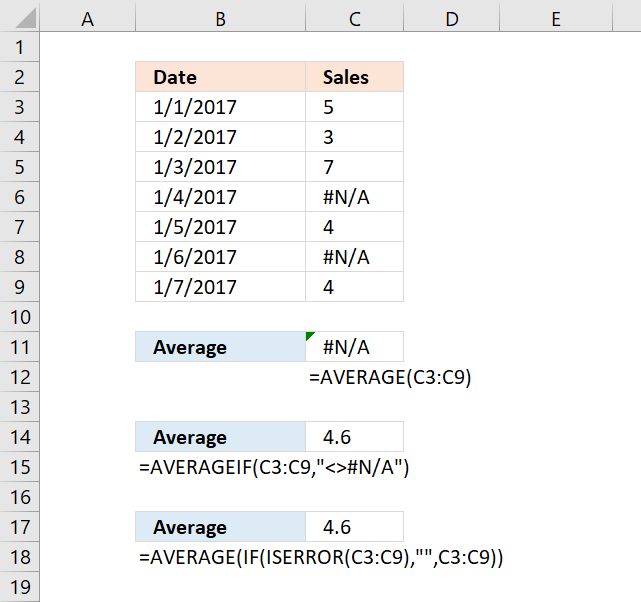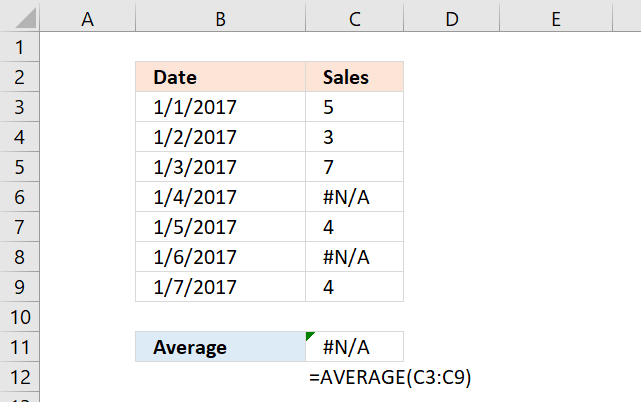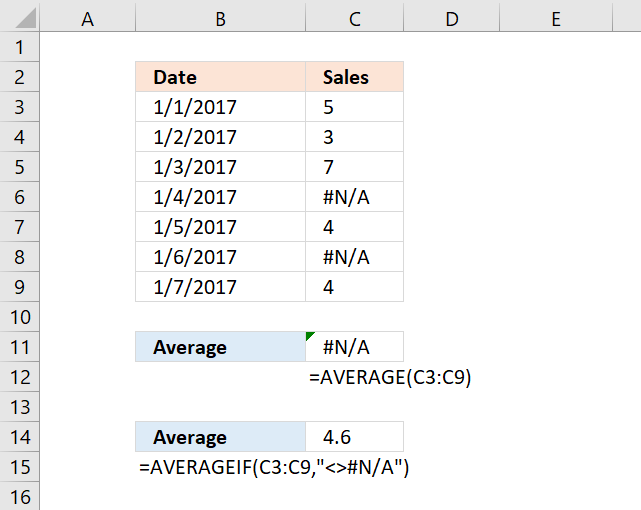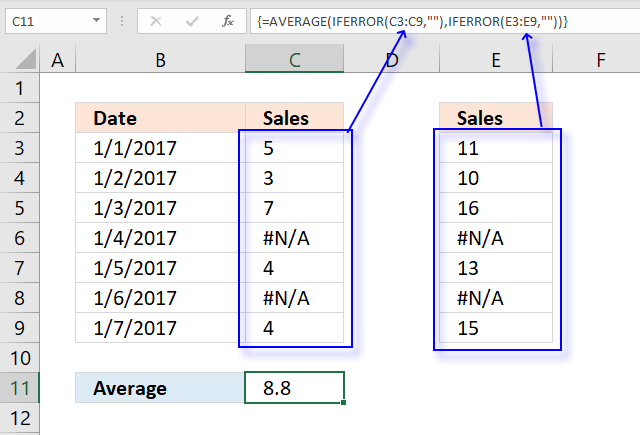Author: Oscar Cronquist Article last updated on December 22, 2021The AVERAGE function ignores empty cells, text values, and boolean values automatically, however, it doesn't handle error values. The AVERAGE function returns an error if the data contains at least one error value.

This article describes a few different workarounds to this problem based on the Excel version you are using. Cell range C3:C9 contains some random numbers and a few #N/A errors, I will use this data to demonstrate a few formulas that can help you out.

## 1. AVERAGE function can't handle errors#N/A error is sometimes used to show gaps in charts, however, the AVERAGE function can't handle errors, shown in C11 in the picture above.

Formula in cell C11:

=AVERAGE(C3:C9)

### Evaluate formula

#### Step 1 - Populate function

The AVERAGE function calculates the average of numbers in a cell range. In other words, the sum of a group of numbers and then divided by the count of those numbers.

AVERAGE(number1[number2], ...)

AVERAGE(C3:C9)

becomes

AVERAGE({5; 3; 7; #N/A; 4; #N/A; 4})

Note that cell reference C3:C9 evaluates to an array with a beginning and ending curly bracket. Values are delimited by a semicolon meaning the values are arranged horizontally.

Your Excel software may show something else than the semicolon, it is determined by your regional settings.

Notice the error value #N/A is displayed in two locations in the array, they correspond to the values in cell C3:C9.

#### Step 2 - Calculate the average

AVERAGE({5; 3; 7; #N/A; 4; #N/A; 4})

returns #N/A. This shows that the AVERAGE function can't ignore error values, you need to somehow handle this using other functions.

## 2. AVERAGE ignore NA() - Excel 2007 and later versionsThere is an easy workaround, the AVERAGEIF function allows you to ignore #N/A errors. It was introduced in Excel 2007.

=AVERAGEIF(C3:C9,"<>#N/A")

### Explaining formula

#### Step 1 - Populate arguments

The AVERAGEIF function returns the average of cell values that are valid for a given condition.

AVERAGEIF(range, criteria, [average_range])

range - C3:C9
criteria - "<>#N/A"
[average_range] - empty

The larger and smaller than signs are the same as "not equal to".

#### Step 2 - Evaluate AVERAGEIF function

AVERAGEIF(C3:C9,"<>#N/A")

returns 4.6

## 3. AVERAGE ignore NA() - earlier versionsIf you have an Excel version earlier than 2007, the ISERROR function works just as fine. The downside is that you need to enter the formula as an array formula.

Array formula in cell C17:

=AVERAGE(IF(ISERROR(C3:C9),"",C3:C9))

### 3.1 How to enter an array formula

To enter an array formula, type the formula in a cell then press and hold CTRL + SHIFT simultaneously, now press Enter once. Release all keys.

The formula bar now shows the formula with a beginning and ending curly bracket telling you that you entered the formula successfully. Don't enter the curly brackets yourself.

### 3.2 Explaining formula

#### Step 1 - Identify error values

The ISERROR function returns TRUE if a value is an error value.

ISERROR(value)

ISERROR(C3:C9)

becomes

ISERROR({5; 3; 7; #N/A; 4; #N/A; 4})

and returns

{FALSE; FALSE; FALSE; TRUE; FALSE; TRUE; FALSE}

#### Step 2 - Replace FALSE with corresponding number

The IF function returns one value if the logical test is TRUE and another value if the logical test is FALSE.

IF(logical_test, [value_if_true], [value_if_false])

IF(ISERROR(C3:C9),"",C3:C9)

becomes

IF({FALSE; FALSE; FALSE; TRUE; FALSE; TRUE; FALSE},"",C3:C9)

becomes

IF({FALSE; FALSE; FALSE; TRUE; FALSE; TRUE; FALSE},"",{5; 3; 7; #N/A; 4; #N/A; 4})

and returns

{5; 3; 7; ""; 4; ""; 4}.

#### Step 3 - Calculate average

The AVERAGE function calculates the average of numbers in a cell range. In other words, the sum of a group of numbers and then divided by the count of those numbers.

AVERAGE(number1[number2], ...)

AVERAGE(IF(ISERROR(C3:C9),"",{5; 3; 7; #N/A; 4; #N/A; 4}))

becomes

AVERAGE({5; 3; 7; ""; 4; ""; 4})

and returns 4.6

## 4. AVERAGE ignore #N/A for non-contiguous cell rangesThe image above demonstrates a formula that calculates an average based on two non-contiguous ranges and ignores error values.

Array formula in cell C11:

=AVERAGE(IFERROR(C3:C9,""),IFERROR(E3:E9,""))

### 4.1 Explaining array formula in cell C11

#### Step 1 - Handle errors in C3:C9

The IFERROR function lets you catch most errors in Excel formulas, it lets you specify a new value replacing the old error value.
IFERROR(value, value_if_error)

IFERROR(C3:C9,"")

becomes

IFERROR({5; 3; 7; #N/A; 4; #N/A; 4}, "")

and returns

{5; 3; 7; ""; 4; ""; 4}.

#### Step 2 - Handle errors in E3:E9

IFERROR(E3:E9,"")

becomes

IFERROR({11; 10; 16; #N/A; 13; #N/A; 15}, "")

and returns

{11; 10; 16; ""; 13; ""; 15}

#### Step 3 - Calculate average

The AVERAGE function calculates the average of numbers in a cell range. In other words, the sum of a group of numbers and then divided by the count of those numbers.

AVERAGE(number1[number2], ...)

AVERAGE(IFERROR(C3:C9,""),IFERROR(E3:E9,""))

becomes

AVERAGE({5; 3; 7; ""; 4; ""; 4}, {11; 10; 16; ""; 13; ""; 15})

and returns 8.8